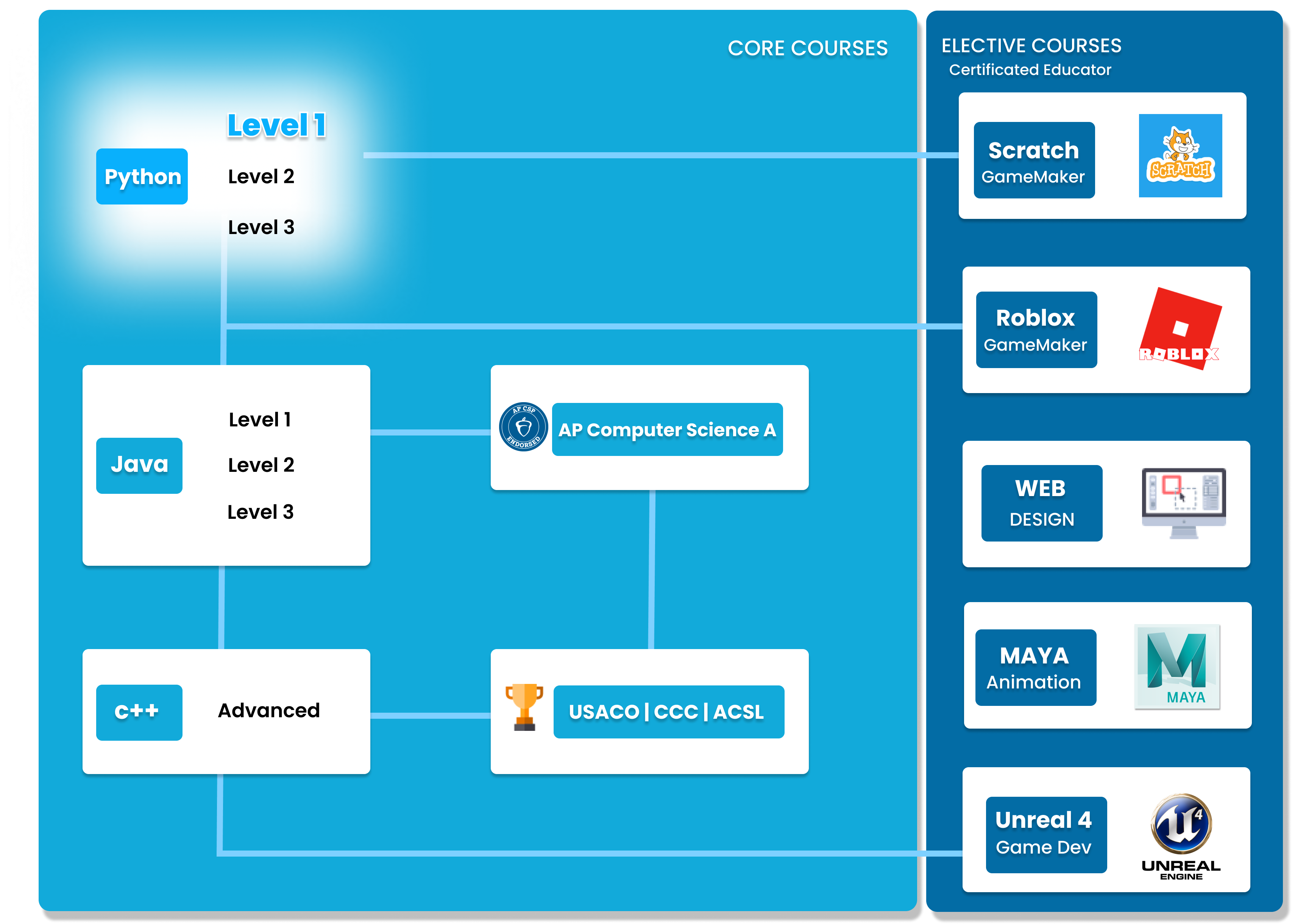# Python Level 1

Designed for beginners, this level teaches the basic fundamentals and design principles of Python, with the help of Turtle graphics and PyGame. This includes: variables, conditional statements, loop basics, and functions. These concepts are transferable to any other programming language. Throughout the course, students will create projects in order to apply the concepts they have learned, and to solidify their knowledge.##### Who is this course for
Beginner Students: This course is for students with no previous Computer Science experience.
This course typically takes 3-6 months to complete. This depends on the student's experience and how fast they can master the concepts and the knowledge. Our instructors move at the pace of the student, it may take extra time for some students to reinforce what they have learned.
##### Curriculum
###### Chapter 1: Variables, Operators and InputStudents will learn about the basic variables: Integers and Floats. Additionally, they will learn how to print to the screen and use mathematical operators, in order to create a Calculator project!
###### Chapter 2: Input and More Variableswork with Strings and Boolean variables, and learn how to take user input. Students will use these to create a Greeting Program.
###### Chapter 3: Drawing with TurtleStudents will learn how to use the concepts learned in chapters 1 and 2, and use it to create drawings using Python with Turtle! They will create a beautiful house drawing or a drawing of their choice.
###### Chapter 4: Conditionals Part 1Students will understand boolean expressions, and how to use them with if and else statements in order to create dynamic programs! At the end of the chapter, students will use this to create a Quiz project.
###### Chapter 5: Conditionals Part 2Chapter 5 dives deeper into conditionals, featuring nested if statements and using logical operators. This allows for more modular and clean code! At the end of the chapter, students will create a Categorizing project.
###### Chapter 6: Decisions with TurtleUsing what they've learned in Chapters 4 and 5, students will create more dynamic Turtle drawings. Additionally, we will talk about coordinates, and manipulating the turtle across the 2d canvas.
###### Chapter 7: Loops Part 1Students will learn how to loop things using a while loop. At the end of the chapter, students will create a final project.
###### Chapter 8: ListsChapter 8 focuses on the list data structure. Students will learn how to create a list, and modify and remove elements.
###### Chapter 9: Loops Part 2After learning lists, students will learn how to use the for loop, how to iterate over sequences, and understand its differences from the while loop.
###### Chapter 10: Fireworks with TurtleChapter 10 uses all the concepts learned in the previous chapters and introduces the random module, in order to create a fireworks animation project.
###### Chapter 11: Functions Part 1Students will be introduced to void functions, how to define functions, and how to call them.
###### Chapter 12: Functions Part 2Chapter 12 dives deeper into functions. Students will learn about non-void functions, return values, and parameters and arguments.
###### Chapter 13: Events with TurtleStudents will wrap up turtle by creating a turtle program that responds to user events. The turtle will follow inputted commands and listen for keyboard events.
###### Chapter 14: Coin GameTo wrap up Level 1, students will create a Coin Game using Pygame. This allows students to utilize what they've learned so far and checks their understanding in a fun and creative way. At the end of the chapter, students will have created a fully customized Coin Game!

### You Might Also Be Interested In Our Elective Courses##### Introduction to Number Theory
AGES 10 - 15

This course is designed to build the basics of a complicated mathematical field. It is designed to be a complete introduction to the fundamental concepts of number theory for students in grades 7-10.

The class covers topics such as primes and composites; multiples, divisors, and divisibility; prime factorization and its uses; modular arithmetic and more.##### Introduction to Geometry
AGES 10 - 15

Students in grades 6-10 will build a solid understanding of more advanced geometry concepts in this course. It is designed to expand on the standard high school geometry curriculum and to challenge students with new concepts and problems.

Topics include congruent and similar triangles, circles, 3D geometry, and introductory trigonometry.##### Introduction to Counting and Probability
AGES 10 - 15

To build on the concepts from the Introduction level course, students will build on their Counting and Probability knowledge.

A thorough introduction for students in grades 7-10 to counting and probability topics such as permutations, combinations, Pascal's triangle, geometric probability, basic combinatorial identities, the Binomial Theorem, Pigeonhole Principle Conditional, Probability Graph Theory and more.